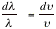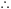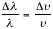How to Do Physics Derivations

When learning a derivation, first write out (in a sentence) each step.  It will help you follow the logic and it will help you to develop a style and framework for relations that you will later derive on your own.

Here is an example for the derivation of Euler's relation,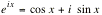:

1.  Write out the general form of the Maclaurin series.

2.  Use the Maclaurin series to expand: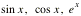.

3.  Use the Maclaurin series to expand4.  Rearrange the terms in the expansion of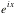grouping the real terms together and the imaginary terms together.

5.  Compare the expression ofwith the expansions of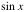and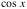and rewriteas a function ofand.

The derivation will look like this:

Show that.

The general form of the Maclaurin series is: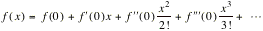(1)

Using (1) to expandgives(2)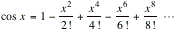(3)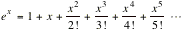Using (1) to expand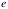to an imaginary power, where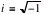gives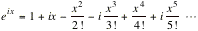(4)

Rearranging (4) we have:Comparing this with (2) and (3) gives: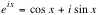Here is another example:

Show that1.  Write down the equation showing the relationship of wavelength, frequency and the speed of light.

2.  Take the magnitude of the derivative with respect to n .

3.  Separateand move one of the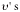ofover to the other side.

4.  Substitute the relationship of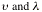for theon the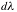side of the equation.

The actual derivation will look like this:

Show that.

For light waves,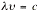.                                                                     (1)

Then the magnitude of the derivative is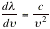and rearranging gives.                                                 (2)

Using (1) in (2) gives